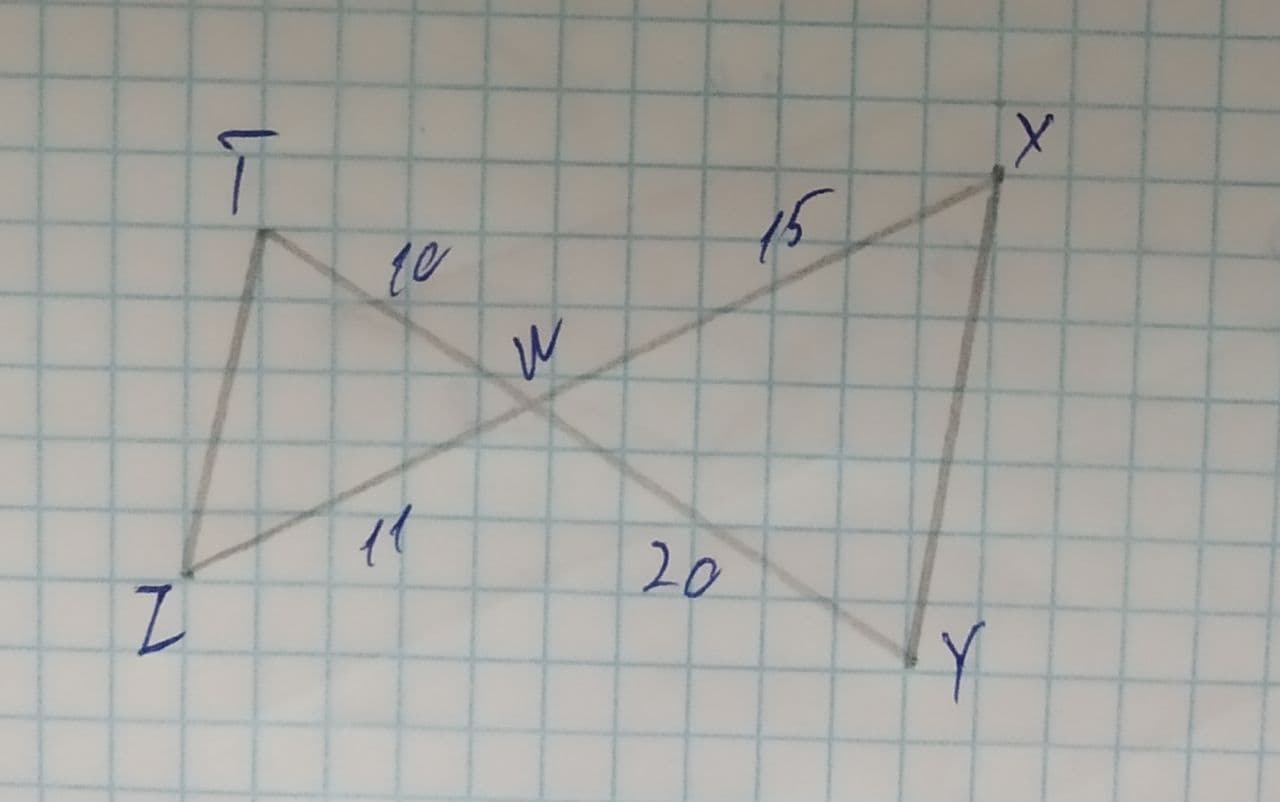Question# Are the triangle similar, if so write a similarity statement.

Similarity
ANSWEREDAre the triangle similar, if so write a similarity statement.2021-08-05

Step 1
If two triangles have two pairs of sides in the same ratio and the included angles are also equal, then the triangles are similar.
Step 2
Now, In $$\displaystyle\triangle{T}{W}{Z}\ {\quad\text{and}\quad}\ \triangle{X}{W}{Y}$$
$$\displaystyle\angle{T}{W}{Z}=\angle{X}{W}{Y}$$
$$\displaystyle{\frac{{{T}{W}}}{{{X}{W}}}}={\frac{{{10}}}{{{15}}}}={\frac{{{2}}}{{{3}}}}$$
$$\displaystyle{\frac{{{Z}{W}}}{{{Y}{W}}}}={\frac{{{11}}}{{{20}}}}\ne{\frac{{{T}{W}}}{{{X}{W}}}}$$
Therefore, these triangles are not similar.